# 4.6 Second-order filters

 Page 1 / 1
Summarizes second order lowpass, bandpass, and highpass fitlers.

## Second-order lowpass filters

The second-order lowpass filter has system function

$H\left(s\right)=\frac{{\Omega }_{n}^{2}}{{s}^{2}+2\zeta {\Omega }_{n}s+{\Omega }_{n}^{2}}$

where ${\Omega }_{n}$ is the undamped natural frequency , and $\zeta$ is the damping ratio . The undamped natural frequency and damping ratio are properties of the physical devices used to implement the second-order filter (capacitors, inductors, and resistors, in the case of an electrical circuit). It so happens that the damping ratio satisfies $\zeta \ge 0$ . Using the formula for the roots of a quadratic polynomial, the two poles of $H\left(s\right)$ are easily found to be

$\begin{array}{cc}\hfill {s}_{1}& =-\zeta {\Omega }_{n}+{\Omega }_{n}\sqrt{{\zeta }^{2}-1}\hfill \\ \hfill {s}_{2}& =-\zeta {\Omega }_{n}-{\Omega }_{n}\sqrt{{\zeta }^{2}-1}\hfill \end{array}$

There are three possible sets of poles that are categorized as follows:

1. Overdamped: the poles are real and distinct. This occurs if $\zeta >1$ . In this case the impulse response is given by:
$h\left(t\right)=\frac{{\Omega }_{n}}{2\sqrt{{\zeta }^{2}-1}}{e}^{{s}_{1}t}u\left(t\right)-\frac{{\Omega }_{n}}{2\sqrt{{\zeta }^{2}-1}}{e}^{{s}_{2}t}u\left(t\right)$
2. Critically damped: corresponds to $\zeta =1$ . The two poles are repeated with,
${s}_{1}={s}_{2}=-\zeta {\Omega }_{n}=-{\Omega }_{n}$
and the impulse response is given by
$h\left(t\right)={\Omega }_{n}^{2}t{e}^{-\zeta {\Omega }_{n}t}u\left(t\right)$
3. Underdamped: corresponds to $0\le \zeta <1$ , giving a pair of complex conjugate poles. In this case, the impulse response is given by
$h\left(t\right)=\frac{{\Omega }_{n}}{\sqrt{1-{\zeta }^{2}}}{e}^{-\zeta {\Omega }_{n}t}sin\left(\sqrt{1-{\zeta }^{2}},{\Omega }_{n},t\right)u\left(t\right)$
Note that in the underdamped case, the magnitude of the poles is $|{s}_{1}|=|{s}_{2}|={\Omega }_{n}$ , and when $\zeta =0$ , the two poles are on the imaginary axis which corresponds to an impulse response that is a pure sinusoid and the system is unstable.

A root locus diagram shows the paths that the poles of $H\left(s\right)$ would take as the damping ratio is decreased from some number greater than 1 down to 0, and is shown in [link] .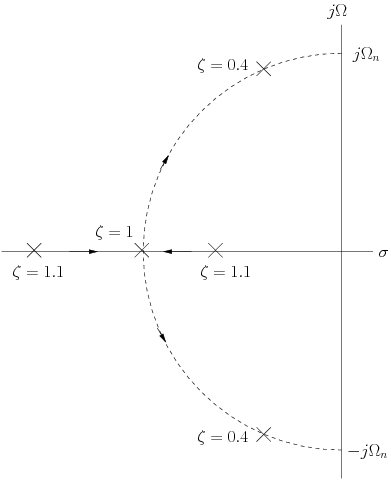Root locus of second-order lowpass filter having Ω n = 10 .

The frequency response of the second-order lowpass filter can be found using the substitution ${H\left(j\Omega \right)=H\left(s\right)|}_{s=j\Omega }$ , giving

$H\left(j,\Omega \right)=\frac{{\Omega }_{n}^{2}}{{\Omega }_{n}^{2}-{\Omega }^{2}+j2\zeta {\Omega }_{n}\Omega }$

The frequency response magnitude is shown in [link] for ${\Omega }_{n}=10$ and several values of $\zeta$ . Note that for the underdamped case, there is a resonance or peak that would be expected to maximize at the undamped natural frequency ${\Omega }_{n}$ , when $\zeta =0$ .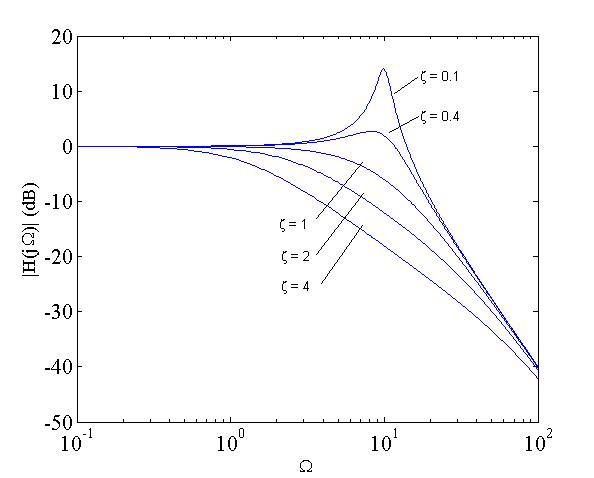Frequency response of second-order lowpass filter with Ω n = 10 and several values of ζ .

We also observe that for the critically damped case, since

$H\left(s\right)=\frac{{\Omega }_{n}^{2}}{{\left(s,+,{\Omega }_{n}\right)}^{2}}$

setting $s=j\Omega$ gives $\left|H,\left({\Omega }_{n}\right)\right|=\frac{1}{2}$ . Moreover, it is clear that for $\Omega <{\Omega }_{n}$ , $\left|H,\left({\Omega }_{n}\right)\right|>\frac{1}{2}$ and for $\Omega >{\Omega }_{n}$ , $\left|H,\left({\Omega }_{n}\right)\right|<\frac{1}{2}$ . These ideas will be used later when we discuss Bode plots.

## Second-order filter implementation

Second order filters can be implemented using either passive or active circuit elements. We will consider the series RLC circuit shown in [link] . A lowpass filter results by taking the filter output to be the voltage across the capacitor. Using voltage division, the system function is easily found to be

$\begin{array}{cc}\hfill {H}_{lp}\left(s\right)& =\frac{{V}_{lp}\left(s\right)}{{V}_{i}\left(s\right)}\hfill \\ & =\frac{\frac{1}{LC}}{{s}^{2}+\frac{R}{L}s+\frac{1}{LC}}\hfill \end{array}$

Comparing [link] with [link] we find that the undamped natural frequency is ${\Omega }_{n}=\frac{1}{\sqrt{LC}}$ , while $2\zeta {\Omega }_{n}=\frac{R}{L}$ , giving the attenuation coefficient:

$\alpha \equiv \zeta {\Omega }_{n}=\frac{R}{2L}$

A second-order highpass filter is implemented by taking the output of the filter to be the inductor voltage in the series RLC circuit. The resulting system function is

$\begin{array}{cc}\hfill {H}_{hp}\left(s\right)& =\frac{{V}_{hp}\left(s\right)}{{V}_{i}\left(s\right)}\hfill \\ & =\frac{{s}^{2}}{{s}^{2}+\frac{R}{L}s+\frac{1}{LC}}\hfill \end{array}$

So in terms of the undamped natural frequency and damping ratio, the second-order highpass filter is given by

${H}_{hp}\left(s\right)=\frac{{s}^{2}}{{s}^{2}+2\zeta {\Omega }_{n}s+{\Omega }_{n}^{2}}$

The graph of the frequency response magnitude of this filter is shown in [link] for several damping ratios and ${\Omega }_{n}=10$ .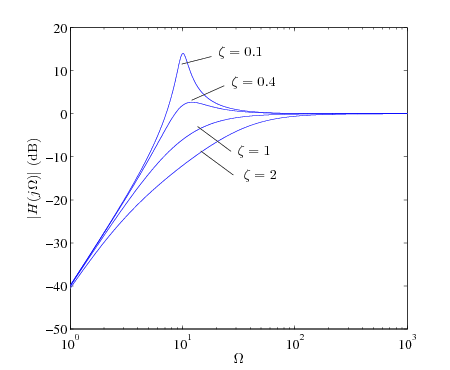Frequency response of second-order highpass filter with Ω n = 10 and several values of ζ .

Not surprisingly, a second-order bandpass filter results by taking the output of the filter be the resistor voltage in the series RLC circuit. The system function is then

$\begin{array}{cc}\hfill {H}_{bp}\left(s\right)& =\frac{{V}_{bp}\left(s\right)}{{V}_{i}\left(s\right)}\hfill \\ & =\frac{\frac{R}{L}s}{{s}^{2}+\frac{R}{L}s+\frac{1}{LC}}\hfill \end{array}$

This can be expressed in terms of $\zeta$ and ${\Omega }_{n}$ as

${H}_{bp}\left(s\right)=\frac{2\zeta {\Omega }_{n}s}{{s}^{2}+2\zeta {\Omega }_{n}s+{\Omega }_{n}^{2}}$

## Frequency response of second-order bandpass filter

Setting $s=j\Omega$ in [link] gives the frequency response of the second-order bandpass filter

${H}_{bp}\left(j\Omega \right)=\frac{j2\zeta {\Omega }_{n}\Omega }{{\Omega }_{n}^{2}-{\Omega }^{2}+j2\zeta {\Omega }_{n}\Omega }$

The magnitude of this frequency response is shown in [link] where ${\Omega }_{n}=10$ .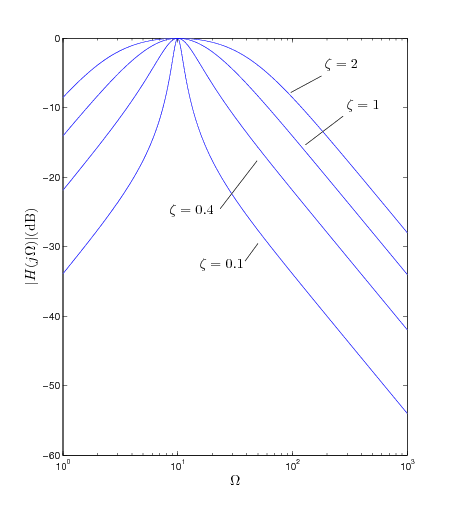Frequency response of second-order bandpass filter with Ω n = 10 and several values of ζ .

Evidently, the frequency response peaks at $\Omega ={\Omega }_{n}$ . To prove this, we can divide the numerator and denominator of [link] by $j2\zeta {\Omega }_{n}\Omega$ , giving

$\begin{array}{cc}\hfill {H}_{bp}\left(j\Omega \right)& =\frac{1}{1-j\frac{{\Omega }_{n}^{2}-{\Omega }^{2}}{2\zeta {\Omega }_{n}\Omega }}\hfill \\ & =\frac{1}{1+j\frac{{\Omega }^{2}-{\Omega }_{n}^{2}}{2\zeta {\Omega }_{n}\Omega }}\hfill \\ \hfill & =\frac{1}{1+jA\left(\Omega \right)}\hfill \\ \hfill \end{array}$

where

$A\left(\Omega \right)=\frac{{\Omega }^{2}-{\Omega }_{n}^{2}}{2\zeta {\Omega }_{n}\Omega }$

It is easy to check that $\left|A,\left(,{\Omega }_{n},\right)\right|=0$ and $\left|A,\left(,\Omega ,\right)\right|>0,\Omega \ne {\Omega }_{n}$ . Therefore $\left|{H}_{bp},\left(j\Omega \right)\right|$ does in fact peak at $\Omega ={\Omega }_{n}$ .

The bandwidth of bandpass filters is typically measured as the difference between the two frequencies, ${\Omega }_{2}$ and ${\Omega }_{1}$ , at which the gain has dropped by 3 decibels from its peak of 0 dB. A diagram of this is shown in [link] .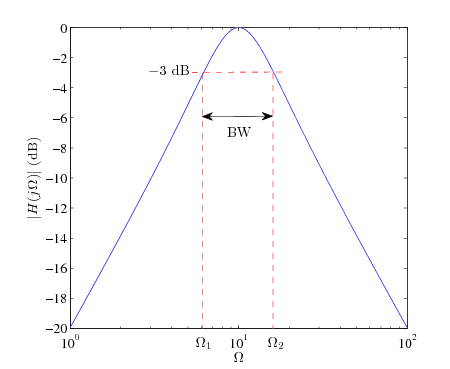Definition of bandpass filter bandwidth, B W = Ω 2 - Ω 1 .

Formulas for ${\Omega }_{1}$ and ${\Omega }_{2}$ can be found by solving

$A\left(\Omega \right)=±1$

since if $A\left(\Omega \right)=±1$ , we get $\left|H,\left(,j,\Omega ,\right)\right|=\frac{1}{\sqrt{2}}$ . Solving the quadratic equation $A\left(\Omega \right)=1$ gives two solutions:

${\stackrel{˜}{\Omega }}_{1}=\zeta {\Omega }_{n}+{\Omega }_{n}\sqrt{1+{\zeta }^{2}}$
${\stackrel{˜}{\Omega }}_{2}=\zeta {\Omega }_{n}-{\Omega }_{n}\sqrt{1+{\zeta }^{2}}$

while solving $A\left(\Omega \right)=-1$ gives two additional solutions

${\stackrel{˜}{\Omega }}_{3}=-\zeta {\Omega }_{n}+{\Omega }_{n}\sqrt{1+{\zeta }^{2}}$
${\stackrel{˜}{\Omega }}_{4}=-\zeta {\Omega }_{n}-{\Omega }_{n}\sqrt{1+{\zeta }^{2}}$

Since ${\Omega }_{n}\sqrt{1+{\zeta }^{2}}>\zeta {\Omega }_{n}$ , we pick the two positive solutions as the “corner” frequencies:

${\Omega }_{1}=-\zeta {\Omega }_{n}+{\Omega }_{n}\sqrt{1+{\zeta }^{2}}$
${\Omega }_{2}=\zeta {\Omega }_{n}+{\Omega }_{n}\sqrt{1+{\zeta }^{2}}$

The two negative frequencies are just the negatives of the two positive frequencies (recall that $\left|H,\left(,j,\Omega ,\right)\right|$ has even symmetry). So the bandwidth is $BW={\Omega }_{2}-{\Omega }_{1}=2\zeta {\Omega }_{n}$ . It can also be readily verified that ${\Omega }_{n}=\sqrt{{\Omega }_{1}{\Omega }_{2}}$ , that is, the center frequency of the bandpass filter is the geometric mean of the two corner frequencies. The qualify factor , ${Q}_{o}$ is defined as

${Q}_{o}=\frac{{\Omega }_{n}}{BW}$

and is a measure of the narrowness of the filter bandwidth, with respect to its center frequency. It can be seen that ${Q}_{o}=\frac{1}{2\zeta }$ .

are nano particles real
yeah
Joseph
Hello, if I study Physics teacher in bachelor, can I study Nanotechnology in master?
no can't
Lohitha
where we get a research paper on Nano chemistry....?
nanopartical of organic/inorganic / physical chemistry , pdf / thesis / review
Ali
what are the products of Nano chemistry?
There are lots of products of nano chemistry... Like nano coatings.....carbon fiber.. And lots of others..
learn
Even nanotechnology is pretty much all about chemistry... Its the chemistry on quantum or atomic level
learn
da
no nanotechnology is also a part of physics and maths it requires angle formulas and some pressure regarding concepts
Bhagvanji
hey
Giriraj
Preparation and Applications of Nanomaterial for Drug Delivery
revolt
da
Application of nanotechnology in medicine
has a lot of application modern world
Kamaluddeen
yes
narayan
what is variations in raman spectra for nanomaterials
ya I also want to know the raman spectra
Bhagvanji
I only see partial conversation and what's the question here!
what about nanotechnology for water purification
please someone correct me if I'm wrong but I think one can use nanoparticles, specially silver nanoparticles for water treatment.
Damian
yes that's correct
Professor
I think
Professor
Nasa has use it in the 60's, copper as water purification in the moon travel.
Alexandre
nanocopper obvius
Alexandre
what is the stm
is there industrial application of fullrenes. What is the method to prepare fullrene on large scale.?
Rafiq
industrial application...? mmm I think on the medical side as drug carrier, but you should go deeper on your research, I may be wrong
Damian
How we are making nano material?
what is a peer
What is meant by 'nano scale'?
What is STMs full form?
LITNING
scanning tunneling microscope
Sahil
how nano science is used for hydrophobicity
Santosh
Do u think that Graphene and Fullrene fiber can be used to make Air Plane body structure the lightest and strongest. Rafiq
Rafiq
what is differents between GO and RGO?
Mahi
what is simplest way to understand the applications of nano robots used to detect the cancer affected cell of human body.? How this robot is carried to required site of body cell.? what will be the carrier material and how can be detected that correct delivery of drug is done Rafiq
Rafiq
if virus is killing to make ARTIFICIAL DNA OF GRAPHENE FOR KILLED THE VIRUS .THIS IS OUR ASSUMPTION
Anam
analytical skills graphene is prepared to kill any type viruses .
Anam
Any one who tell me about Preparation and application of Nanomaterial for drug Delivery
Hafiz
what is Nano technology ?
write examples of Nano molecule?
Bob
The nanotechnology is as new science, to scale nanometric
brayan
nanotechnology is the study, desing, synthesis, manipulation and application of materials and functional systems through control of matter at nanoscale
Damian
Is there any normative that regulates the use of silver nanoparticles?
what king of growth are you checking .?
Renato
how did you get the value of 2000N.What calculations are needed to arrive at it
Privacy Information Security Software Version 1.1a
Good
Got questions? Join the online conversation and get instant answers!

#### Get Jobilize Job Search Mobile App in your pocket Now!By Jonathan LongBy OpenStaxBy OpenStaxBy Dan ArielyBy Brooke DelaneyBy OpenStaxBy OpenStaxBy Rohini AjayBy Joanna SmithbackBy Madison Christian